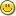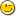### 1. centering display

```integer RM = 50
display("[c:50]", {"This text is centered"})
display("[c:RM]", {"This text is not centered"})
constant VC=graphcst:video_config()
-- or
RM = VC[VC_COLUMNS]
display("[c:RM]", {"This text is not centered"})
```

```              This text is centered
This text is not centered
This text is not centered
```

Is there anyway to use a variable to pass a value to the the display center function?

### 2. Re: centering display

Senator said...
```integer RM = 50
display("[c:50]", {"This text is centered"})
display("[c:RM]", {"This text is not centered"})
constant VC=graphcst:video_config()
-- or
RM = VC[VC_COLUMNS]
display("[c:RM]", {"This text is not centered"})
```

Is there anyway to use a variable to pass a value to the the display center function?

Since it's a string anyway, you could use

```display("[c:" & sprint(RM) & "]", {"This text is not centered"})
```

That's one way.

### 3. Re: centering display

euphoric said...

Since it's a string anyway, you could use

```display("[c:" & sprint(RM) & "]", {"This text is not centered"})
```

That's one way.

Thanks euphoric, works like a charm.### 4. Re: centering display

Senator said...
euphoric said...

Since it's a string anyway, you could use

```display("[c:" & sprint(RM) & "]", {"This text is not centered"})
```

That's one way.

Thanks euphoric, works like a charm.Yeah, but doesn't that say This text is not centered when actually it is centered? scnrOn a slightly more serious note,

```printf(1,">%6s<\n",{"four"}) -- produces ">  four<"
printf(1,">%-6s<\n",{"four"}) -- produces ">four  <"
```

Does anyone any have any suggestion/preference for a format string that would produce "> four <"?
(Obviously, there isn't one, but if I were to implement it what would feel right as the third alternative to "%6s" and "%-6s"? "%|6s"? "%=6s"?)

### 5. Re: centering display

petelomax said...

On a slightly more serious note,

```printf(1,">%6s<\n",{"four"}) -- produces ">  four<"
printf(1,">%-6s<\n",{"four"}) -- produces ">four  <"
```

Does anyone any have any suggestion/preference for a format string that would produce "> four <"?
(Obviously, there isn't one, but if I were to implement it what would feel right as the third alternative to "%6s" and "%-6s"? "%|6s"? "%=6s"?)

I'd recommend "%^6s" or "%+6s".

-Greg

### 6. Re: centering display

ghaberek said...

I'd recommend "%^6s" or "%+6s".

Thanks, unfortunately + is already in use on numbers to show a plus sign on positive values, so using that would mean you cannot centre numbers and/or break existing code.

Is there any preference for splitting say 7 spaces as 4:3 or 3:4?

### 7. Re: centering display

How about "%<6s" for left, "%>6s" for right, and "%|6s" for middle (centre) justification? The'|' suggests dividing space in middle to me.

3/4 or 4/3 - why not just toss a coin?Sasha

### 8. Re: centering display

Quick and Dirty:

```-- add this at the end of std/console.e
public sequence CS --Center text string
public function CenterString(integer RM)
--returns center string for the display routine,
--given RM, the margin width
return "[c:" & sprint(RM) & "]"
end function
-----------------------------------------------------
-- test
sequence text = "this is a test"
CS = CenterString(25)
display(CS,{text})
```

### 9. Re: centering display

Thanks again, euphoric!```--
-- center_display.e
--

include std/text.e
include std/console.e

public procedure center_display(sequence text, integer right_margin)
display("[c:" & sprint(right_margin) & "]",{text})
end procedure

-- test:
center_display("This Text Is Centered",60)
```

### 10. Re: centering display

Just for the record, those changes mentioned above were indeed put into Phix:

```printf(1,">%6s<\n",{"four"}) -- produces ">  four<"
printf(1,">%-6s<\n",{"four"}) -- produces ">four  <"
printf(1,">%=6s<\n",{"four"}) -- produces "> four <"
printf(1,">%=7s<\n",{"four"}) -- produces "> four  <"
printf(1,">%|7s<\n",{"four"}) -- produces ">  four <"
```

### 11. Re: centering display

Blast from the past:

```--------------------------------------------------------------------------
-- formtext.e by Mike Nelson
-- text formatting for DOS/Linux

include graphics.e

public constant ALIGN_LEFT=1
public constant ALIGN_CENTER=2
public constant ALIGN_RIGHT=3

public integer screenColumns,
leftMargin, rightMargin,
tabSize,
textAlignment

public enum l,r
public sequence margin = {0,0}
--
--
public procedure init_text_formatter()
-- initializes screen parameters
-- call after changing video modes
sequence vidconf=video_config()
screenColumns=vidconf[VC_COLUMNS]
leftMargin=1
rightMargin=screenColumns
tabSize=5
textAlignment=ALIGN_LEFT
end procedure
init_text_formatter()  -- first initialization is automatic
--
public function set_margins(integer left,integer right)
-- Sets margins, returns 1 or 0 on invalid margin(s).
-- left=0 or right=0 leaves that margin unchanged
-- a negative value for right indicates moving left
if left<0 then return 0 end if
if right<0 then right=screenColumns+right end if
if right>screenColumns then return 0 end if
if left>right then return 0 end if
if left>0 then leftMargin=left end if
if right>0 then rightMargin=right end if
return 1
end function
--
public function get_margins()
return {leftMargin,rightMargin}
end function
margin = get_margins()
--
public function set_tabsize(integer size)
-- sets tabsize, returns 1 or 0 on invalid size.
if size<0 then return 0 end if
if size>rightMargin-leftMargin+1 then return 0 end if
tabSize=size
return 1
end function
--
public function get_tabsize()
return tabSize
end function
--
public function set_alignment(integer align)
-- ALIGN_LEFT, ALLIGN_CENTER, ALLIGN_RIGHT
--sets alignment, returns 1. Returns 0 on invalid alignment
if find(align, {1,2,3}) then
textAlignment=align
return 1
else
return 0
end if
end function
--
public function get_alignment()
return textAlignment
end function
--
function ltrim(sequence text)
for i=1 to length(text) do
if text[i]!=' ' then
return text[i..length(text)]
end if
end for
return ""
end function

function rtrim(sequence text)
for i=length(text) to 1 by -1 do
if text[i]!=' ' then
return text[1..i]
end if
end for
return ""
end function
--
procedure print_aligned(sequence text,integer width)
sequence lspace,rspace
integer spaces
spaces=width-length(text)
if textAlignment=ALIGN_LEFT then
lspace=""
rspace=repeat(' ',spaces)
elsif textAlignment=ALIGN_CENTER then
lspace=repeat(' ',floor(spaces/2))
rspace=repeat(' ',floor((spaces+1)/2))
else
lspace=repeat(' ',spaces)
rspace=""
end if
puts(1,lspace&text&rspace)
end procedure
--
public procedure print_formatted(sequence text)
--Prints formatted text to screen starting at current postion,
--using the curent margin and alignment settings.

--Output is word-wrapped if possible, hyphenated if not.
--Tabs are expanded to the number of spaces given
--by the current tabsize setting.
--Whitespace is trimmed only at word wraps and hyphenations.
--Assumes text is a valid string.

integer width,offset,index
sequence pos,printText
while 1 do
index=find('\t',text)
if index=0 then exit end if
text=text[1..index-1]&repeat(' ',tabSize)&text[index+1..length(text)]
end while
width=rightMargin-leftMargin+1
pos=get_position()
offset=0
if pos>rightMargin then
puts(1,"\n")
pos=get_position()
end if
if pos<leftMargin then
position(pos,leftMargin)
elsif pos>leftMargin then
offset=pos-leftMargin
end if
index=find('\n',text)
if index=0 then index=length(text)+1 end if
if index<=width-offset+1 then
print_aligned(text[1..index-1],width-offset)
if index<=length(text) then
print_formatted(text[index+1..length(text)])
end if
return
end if
for i=width-offset+1 to 1 by -1 do
if text[i]=' ' then
print_aligned(rtrim(text[1..i-1]),width)
print_formatted(ltrim(text[i+1..length(text)]))
return
end if
end for
print_aligned(text[1..width-offset-1]&'-',width-offset)
if length(text)>=width-offset then
print_formatted(ltrim(text[width-offset..length(text)]))
end if
end procedure
```